Posted: Jan 06, 2016 10:44 pm
As it's next on my to do list, and ties in nicely with THWOTH's statement "As a lick is a base unit of musical information it can be manipulated in two significant ways: by time and by pitch. " it is time to drill into what exactly is musical information.

I've mentioned the concept of entropy as a measure of the amount of musical information in a lick from time to time in the thread and have given brief explanations of what I mean, and some examples, but now it's time to get more specific.

Some of this is review and perhaps very familiar material to some but it may be helpful to start at the beginning.

Claude Shannon introduced the concept that the amount of information being communicated has something to do with the probability of the underlying symbols being sent. (There are some issues with the language, the symbols used to communicate, i.e. "Shaka, When the Walls Fell" has a certain measure of information content when English letters are used to communicate it, but that doesn't measure the actual amount of information that " "Shaka, When the Walls Fell" can actually communicate. Soooo. we're dealing with the symbols being sent not the underlying information content of those symbols.

An overview of information theory can be found here. but this comment should explain all you need to know for this thread.

Shannon, building on work previously done in statistical mechanics, realized that the amount of information being communicated can be calculated to be:So I don't mess up the meaning of this I'll just quote from the Wiki article:

The entropy, H, of a discrete random variable X intuitively is a measure of the amount of uncertainty associated with the value of X when only its distribution is known. So, for example, if the distribution associated with a random variable was a constant distribution, (i.e. equal to some known value with probability 1), then entropy is minimal, and equal to 0. Furthermore, in the case of a distribution restricted to take on a finite number of values, entropy is maximized with a uniform distribution over the values that the distribution takes on.

A simple example should clear up any confusion. We can use Shannon's equation to calculate how many bist we need to code n distinct symbols. This is most familiar with there is an equal probability of each symbol occurring. So, if we want to represents four symbols or states, each with equal probability.

The Entropy (H or E which every you like) = - the sum of (the probability of each symbol) * Log(probability of each symbol)

in our example the probability of each symbol is .25
we want our answer in bits so we use log base 2
log2 .25 = -2
so the sum value for symbol 1) = probability of symbol 1 * Log(probability of symbol1) = .25 * -2 = -.5

and since each of the symbols are the same, the sum is just 4 times that of the first symbol. -.5 *4 = -2
and taking the - in front of the sum into account we get 2 - that's 2 bits are required to communicate four symbols with equal probability.

Of course, things get a bit more tricky to wrap your head around when the probability for the symbols occurring differ - but that's what computers are for.

I'm applying this concept to measure the symbol content of a lick. There are multiple ways this could be done- it depends on how you define the symbols used to communicate music. One could boil down all the information content of a lick to one number, it's information entropy (more or less), but music has several fairly obvious different information properties and it's useful to measure the information content of each of those properties separately. Doing this, I end up with an entropy vector for each lick. The Entropy vector has a pitch component, a rhythm component, and a vertical (simultaneous harmony- multiple pitches played at the same time) component. If you want one number to represent the entire vector you can use the magnitude of the vector.

In short, the more symbol variety of a lick in any of those components the greater that component's entropy. Some examples without the math should clarify this nicely. I'll use my entropy test licks because they are easy to understand.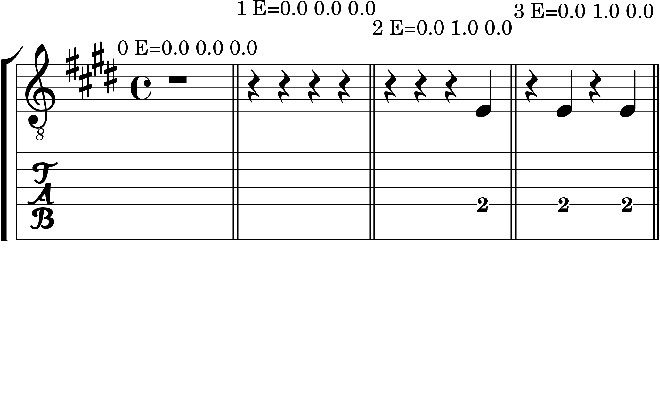The figure above shows four licks, 0-3. An Entropy vector with 3 components is printed above each lick. The components, in order are, pitch, rhythm, vertical harmony.

Lick 0 is just a bar of rest. One symbol, with a probability of 1 is all that is needed to describe it. The log of 1 =0 so it's entropy is 0. In this case there are no pitch and harmony symbols - again 0 entropy.

Lick 1 is musically the same as Lick 0 so we expect and get the same answer.

Lick 2 is effectively composed of two symbols (the three rests could have been written as a dotted half note rest- which is one symbol) and the quarter note. So, we have two symbols with equal probability that's 1 bit of information.

Lick 3 is once again 2 symbols with equal probability.

There is more than one way to factor rests into the entropy calculation. I'm ignoring rests in pitch and vertical entropy and only considering it in rhythm entropy. But I'm open to other ideas on how this should be done.

Some more licks: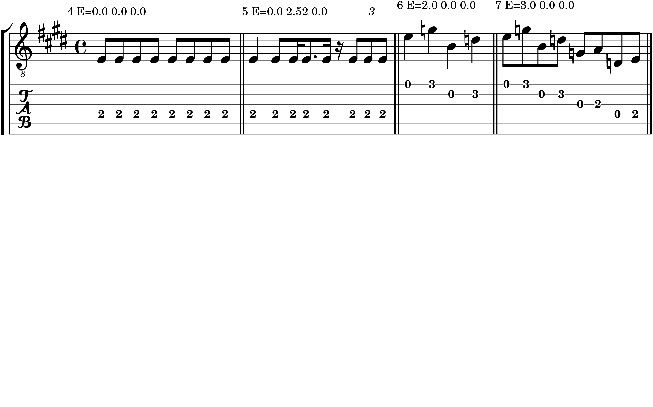Lick 4 is the same note with the same duration always being played. The probability of what symbol is next is 1 so the licks entropy is 0.

Lick 5 has a lot of variety in note duration, this gives it high rhythm entropy but nothing much is happening pitch wise or harmonically.

Lick 6 shows some pitch variety and it's pitch entropy show that, Lick 7 has even more pitch variety and a greater pitch entropy.

Next we get to some examples that show vertical or what I sometimes call simultaneous harmony entropy.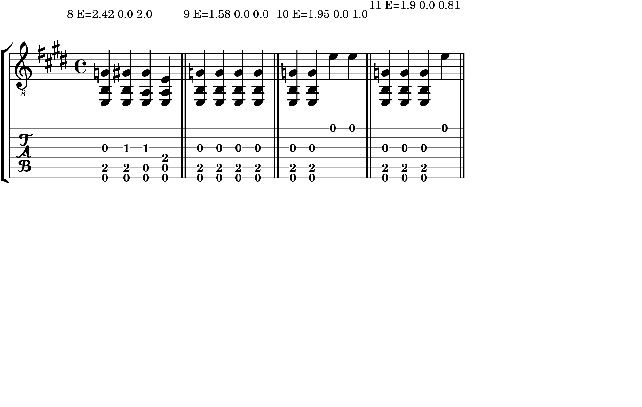To measure vertical entropy I consider different simultaneous note intervals as different symbols. That is, if the chord has a root, Maj3rd, P5 interval content then it gets the same symbol no matter the root. If different chords are played that shows up in the pitch entropy.

Lick 8 has the highest pitch entropy because every chord has different intervals. Like 9 has a perfectly predictable vertical pitch interval so its vertical entropy is 0. Lick 10 has effectively 1 bit of vertical entropy. Lick 11 has lower vertical entropy.

Now all of this is way more tedious to explain than understand and relate to. It can all be said simply as: the greater the pitch entropy the greater the pitch varies, the greater the rhythm entropy the greater the rhythm varies, the greater the vertical entropy the greater the vertical intervals vary.

There are some approximations made here and there in my calculations but they're mostly nits and I won't go into that. And I'm still checking this so there might be a bug here or there- but I think you get the idea.

Now that we have a measure of various information components of licks we can plot a set of licks and see how their information content compares. Below is a plot of the test licks I described above. (The vertical axis is vertical (harmony) entropy)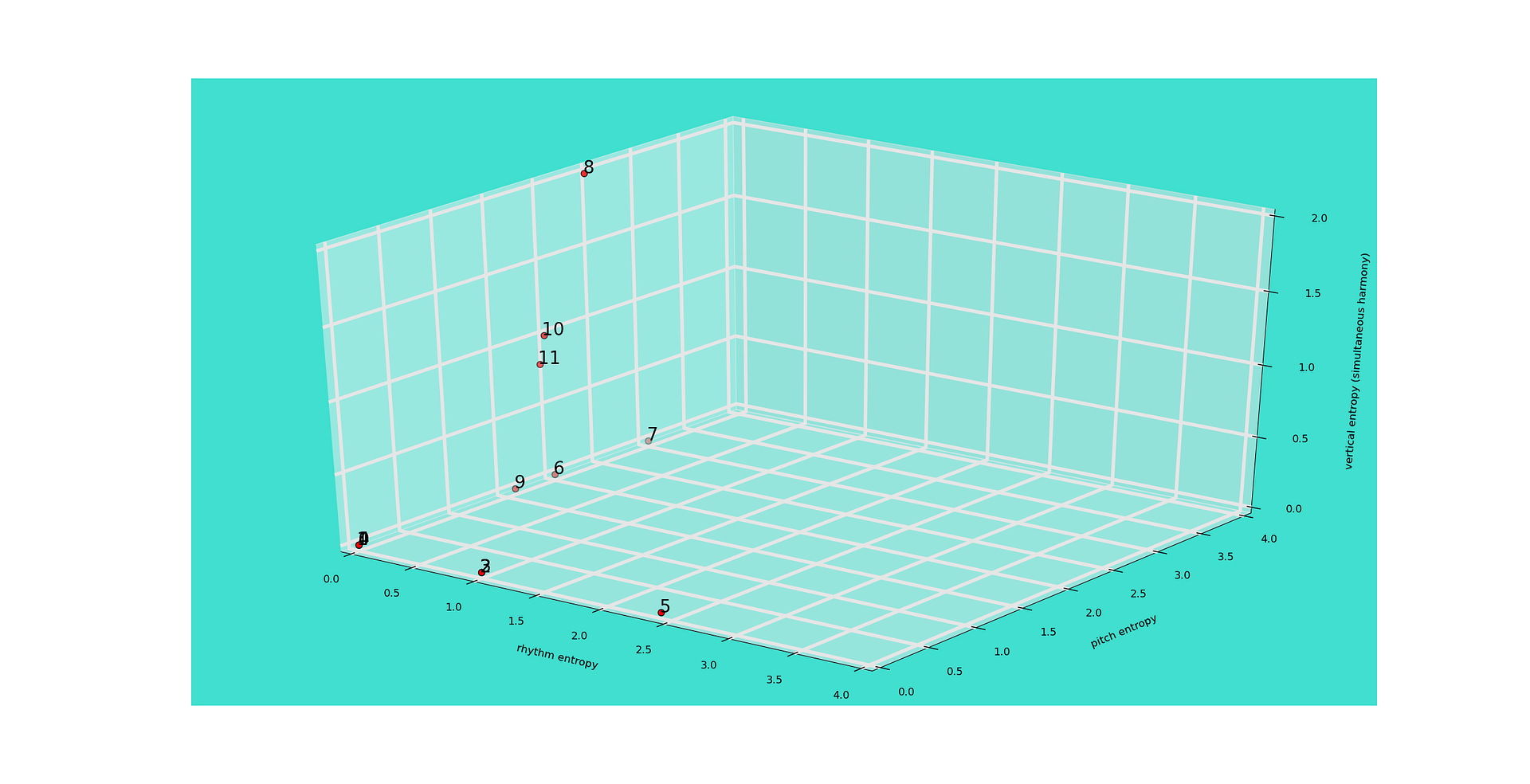And we can plot the founder set of licks that I've been using throughout the thread. I won't post the scores for those licks as I suspect this is already way to tedious but if someone wants it - just ask.

There wasn't a lot happening vertically in those licks.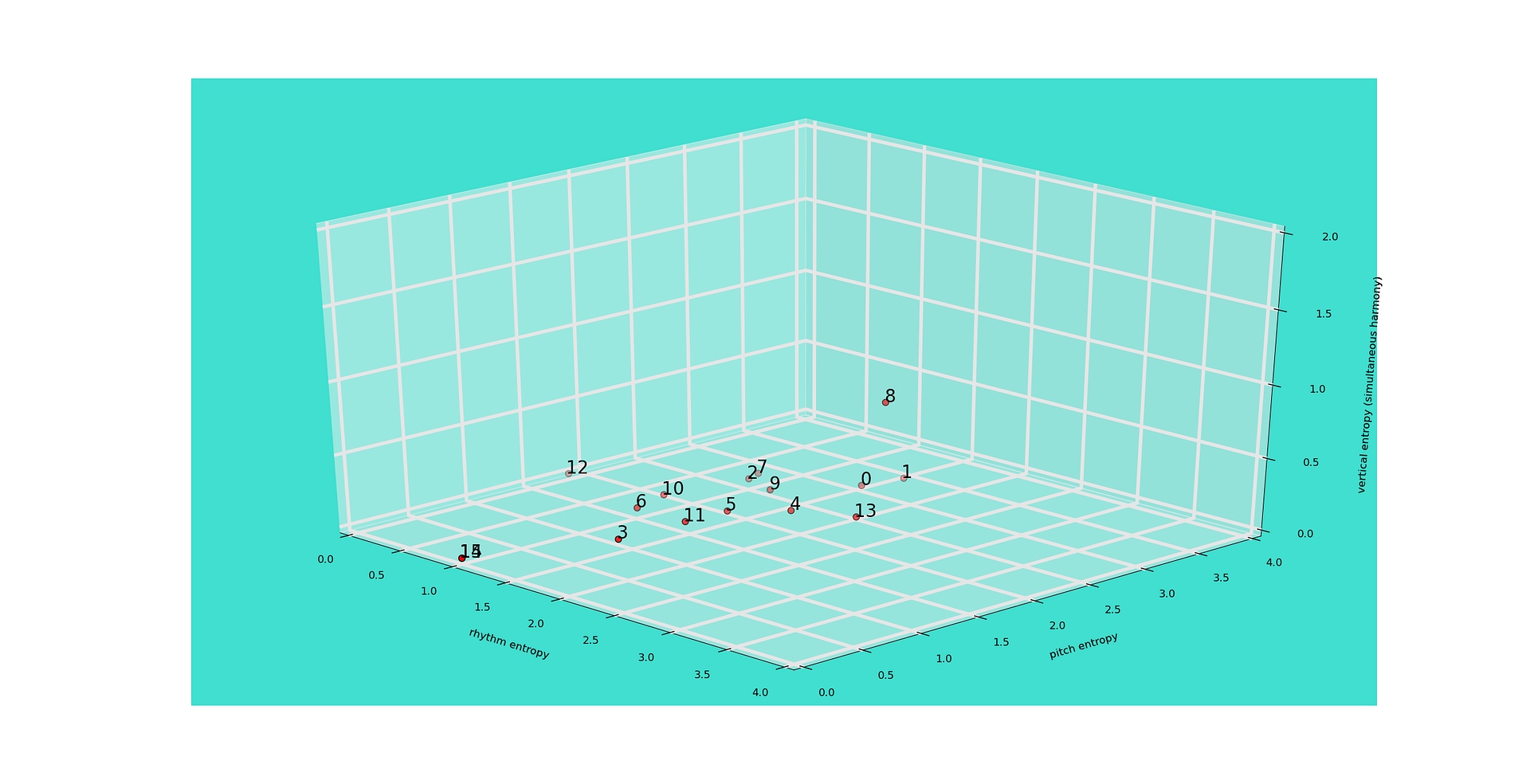And after a couple of generation of mutations of all sorts except no spawning . (resulting in about 700 licks)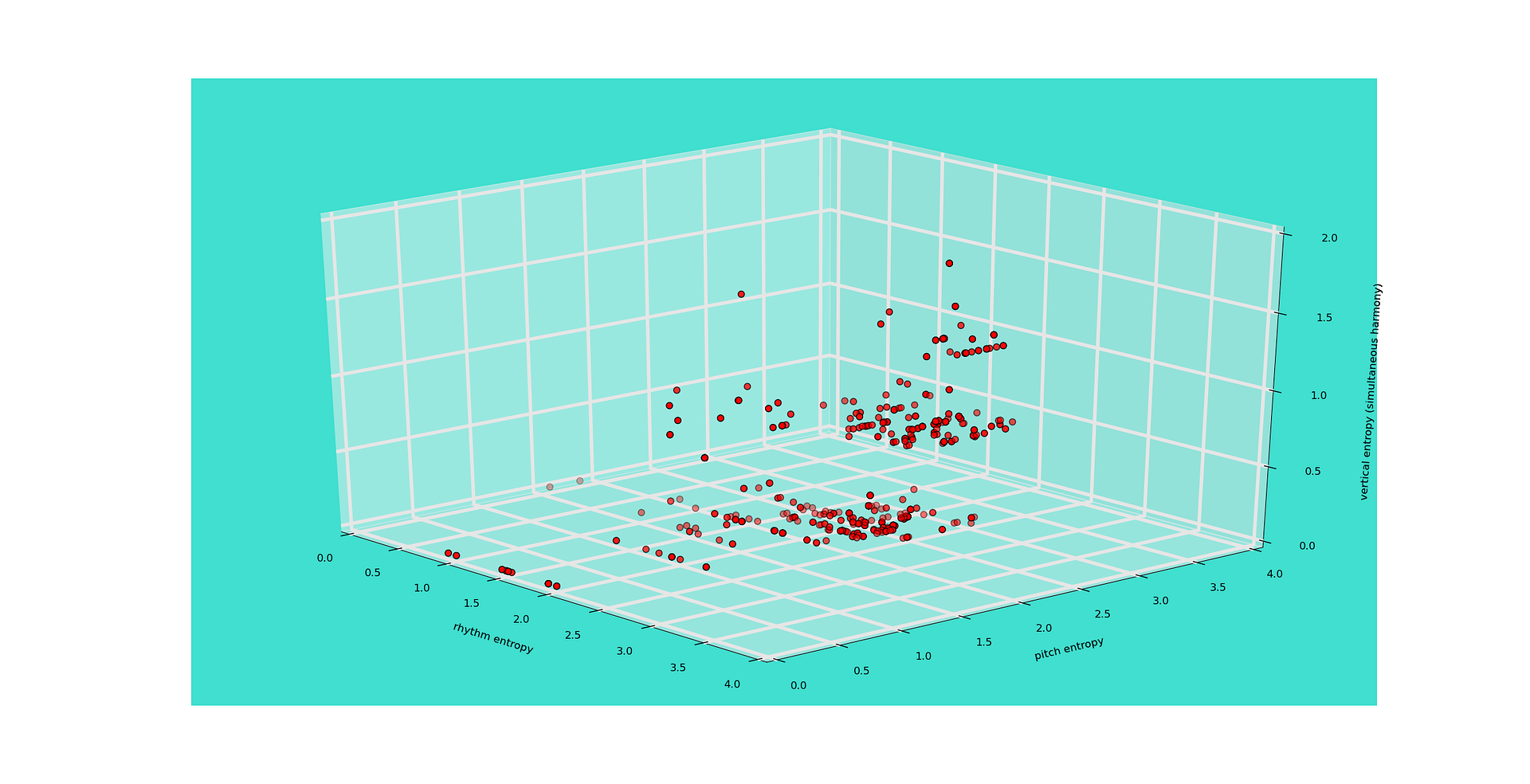After a couple generations with spawning - still about 700 licks.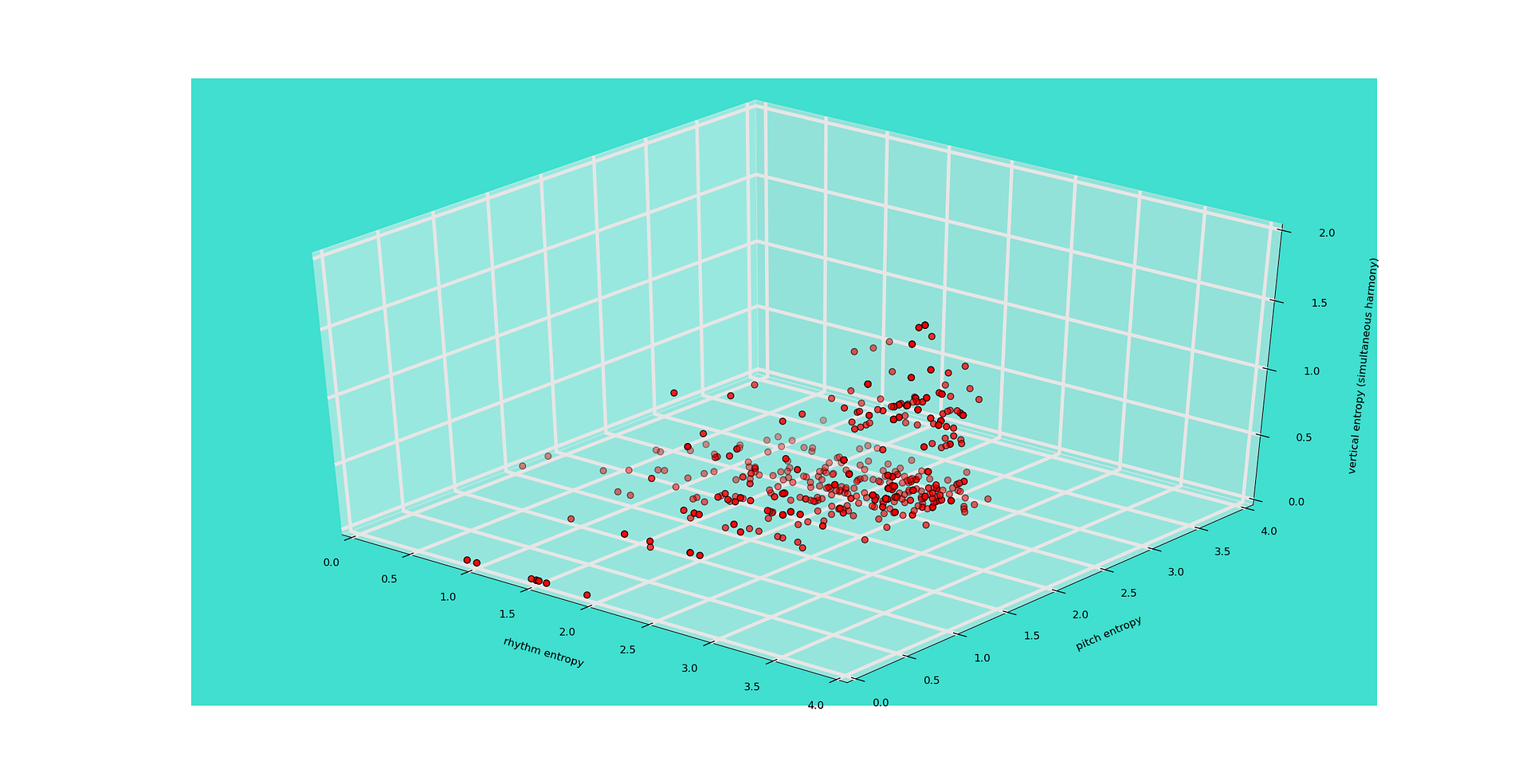And that's my multidimensional entropy calculation. The plan is for it to play a center role as I develop my simulated annealing heuristic based on constructor theory. Briefly, the idea is that one way to guide, that is, one knob of the heuristic, is the definition of a volume in entropy space and a density function. The heuristic will attempt to fill the space with the specified density function, licks with entropies that fall outside the defined volume will not be able to exist.

What else ... There is other information in music that this doesn't cover. ie. dynamics, i.e volume, ways to hit a note, picked, vs hammer ons, but most of what would appear on the score is being taken into consideration. By looking at where a lick falls in my entropy space you should be able to have some idea of what the lick might feel like. Of course knowing the amount of information says nothing about the actual information- so more metrics are needed.

Any questions.